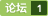matlab程序关于小波变换实例下载 [问题点数：0分]EWT 经验小波变换matlab源程序

matlab实现连续<em>小波变换</em>，仅供参考，互相学习
matlab程序关于小波变换实例

matlab<em>小波变换</em>、离散<em>小波变换</em>的多层分解、主要用于有机质

DWT小波变换MATLAB
DWT<em>小波变换</em>MATLAB 里面有图片 亲测可用

<em>小波变换</em>（wavelet transform，WT）是一种新的变换分析方法，它继承和发展了短时傅立叶变换局部化的思想，同时又克服了窗口大小不随频率变化等缺点，能够提供一...

<em>小波变换</em>的图像处理%MATLAB2维<em>小波变换</em>经典程序 % FWT_DB.M; % 此示意程序用DWT实现二维<em>小波变换</em> % 编程时间2004-4-10，编程人沙威 %%%%%%%%%%%%%%%%%%%%%%%%%%%% %%%%%%%%%%%%%%%%%%%%%%%%%%% clear; clc; T=256; % 图像维数 SUB_T=T/2; % 子图维数 %%%%%%%%%%%%%%%%%%%%%%%%%%%%%%%%% %%%%%%%%%%%%%%%%%%%%%%%%%%%%%%%%% % 1.调原始图像矩阵 load wbarb; % <em>下载</em>图像 f=X; % 原始图像 %%%%%%%%%%%%%%%%%%%%%%%%%%%%%%%% %%%%%%%%%%%%%%%%%%%%%%%%%%%%%%%% % 2.进行二维小波分解 l=wfilters('db10','l'); % db10（消失矩为10)低通分解滤波器冲击响应（长度为20） L=T-length(l); l_zeros=[l,zeros(1,L)]; % 矩阵行数与输入图像一致，为2的整数幂 h=wfilters('db10','h'); % db10（消失矩为10)高通分解滤波器冲击响应（长度为20） h_zeros=[h,zeros(1,L)]; % 矩阵行数与输入图像一致，为2的整数幂 for i=1:T; % 列变换 row(1:SUB_T,i)=dyaddown( ifft( fft(l_zeros).*fft(f(:,i)') ) ).'; % 圆周卷积FFT row(SUB_T+1:T,i)=dyaddown( ifft( fft(h_zeros).*fft(f(:,i)') ) ).'; % 圆周卷积FFT end; for j=1:T; % 行变换 line(j,1:SUB_T)=dyaddown( ifft( fft(l_zeros).*fft(row(j,:)) ) ); % 圆周卷积FFT line(j,SUB_T+1:T)=dyaddown( ifft( fft(h_zeros).*fft(row(j,:)) ) ); % 圆周卷积FFT end; decompose_pic=line; % 分解矩阵 % 图像分为四块 lt_pic=decompose_pic(1:SUB_T,1:SUB_T); % 在矩阵左上方为低频分量--fi(x)*fi(y) rt_pic=decompose_pic(1:SUB_T,SUB_T+1:T); % 矩阵右上为--fi(x)*psi(y) lb_pic=decompose_pic(SUB_T+1:T,1:SUB_T); % 矩阵左下为--psi(x)*fi(y) rb_pic=decompose_pic(SUB_T+1:T,SUB_T+1:T); % 右下方为高频分量--psi(x)*psi(y) %%%%%%%%%%%%%%%%%%%%%%%%%%%%%%%%%%%%%%%%%%% %%%%%%%%%%%%%%%%%%%%%%%%%%%%%%%%%%%%%%%%%%% % 3.分解结果显示 figure(1); colormap(map); subplot(2,1,1); image(f); % 原始图像 title('original pic'); subplot(2,1,2); image(abs(decompose_pic)); % 分解后图像 title('decomposed pic'); figure(2); colormap(map); subplot(2,2,1); image(abs(lt_pic)); % 左上方为低频分量--fi(x)*fi(y) title('\Phi(x)*\Phi(y)'); subplot(2,2,2); image(abs(rt_pic)); % 矩阵右上为--fi(x)*psi(y) title('\Phi(x)*\Psi(y)'); subplot(2,2,3); image(abs(lb_pic)); % 矩阵左下为--psi(x)*fi(y) title('\Psi(x)*\Phi(y)'); subplot(2,2,4); image(abs(rb_pic)); % 右下方为高频分量--psi(x)*psi(y) title('\Psi(x)*\Psi(y)'); %%%%%%%%%%%%%%%%%%%%%%%%%%%%%%%%%%%%%%%%%%% %%%%%%%%%%%%%%%%%%%%%%%%%%%%%%%%%%%%%%%%%%%% % 5.重构源图像及结果显示 % construct_pic=decompose_matrix'*decompose_pic*decompose_matrix; %%%%%%%%%%%%%%%%%%%%%%%%%%%%%%%%%%%%%%%%%%%%%%%%%%%%%%%%%%%%%% l_re=l_zeros(end:-1:1); % 重构低通滤波 l_r=circshift(l_re',1)'; % 位置调整 h_re=h_zeros(end:-1:1); % 重构高通滤波 h_r=circshift(h_re',1)'; % 位置调整 %%%%%%%%%%%%%%%%%%%%%%%%%%%%%%%%%%%%%%%%%%%%%%%%%%%%%%%%%%%%%%% top_pic=[lt_pic,rt_pic]; % 图像上半部分 t=0; for i=1:T; % 行插值低频 if (mod(i,2)==0) topll(i,:)=top_pic(t,:); % 偶数行保持 else t=t+1; topll(i,:)=zeros(1,T); % 奇数行为零 end end; for i=1:T; % 列变换 topcl_re(:,i)=ifft( fft(l_r).*fft(topll(:,i)') )'; % 圆周卷积FFT end; %%%%%%%%%%%%%%%%%%%%%%%%%%%%%%%%%%%%%%%%%%%%%%%%%%%%%%%%%%%%%% bottom_pic=[lb_pic,rb_pic]; % 图像下半部分 t=0; for i=1:T; % 行插值高频 if (mod(i,2)==0) bottomlh(i,:)=bottom_pic(t,:); % 偶数行保持 else bottomlh(i,:)=zeros(1,T); % 奇数行为零 t=t+1; end end; for i=1:T; % 列变换 bottomch_re(:,i)=ifft( fft(h_r).*fft(bottomlh(:,i)') )'; % 圆周卷积FFT end; construct1=bottomch_re+topcl_re; % 列变换重构完毕 %%%%%%%%%%%%%%%%%%%%%%%%%%%%%%%%%%%%%%%%%%%%%%%%%%%%%%%%%%%% left_pic=construct1(:,1:SUB_T); % 图像左半部分 t=0; for i=1:T; % 列插值低频 if (mod(i,2)==0) leftll(:,i)=left_pic(:,t); % 偶数列保持 else t=t+1; leftll(:,i)=zeros(T,1); % 奇数列为零 end end; for i=1:T; % 行变换 leftcl_re(i,:)=ifft( fft(l_r).*fft(leftll(i,:)) ); % 圆周卷积FFT end; %%%%%%%%%%%%%%%%%%%%%%%%%%%%%%%%%%%%%%%%%%%%%%%%%%%%%%%%%%%% right_pic=construct1(:,SUB_T+1:T); % 图像右半部分 t=0; for i=1:T; % 列插值高频 if (mod(i,2)==0) rightlh(:,i)=right_pic(:,t); % 偶数列保持 else rightlh(:,i)=zeros(T,1); % 奇数列为零 t=t+1; end end; for i=1:T; % 行变换 rightch_re(i,:)=ifft( fft(h_r).*fft(rightlh(i,:)) ); % 圆周卷积FFT end; %%%%%%%%%%%%%%%%%%%%%%%%%%%%%%%%%%%%%%%%%%%%%%%%%%%%%%%%%% construct_pic=rightch_re+leftcl_re; % 重建全部图像 %%%%%%%%%%%%%%%%%%%%%%%%%%%%%%%%%%%%%%%%%%%%%%%%%%%% % 结果显示 figure(3); colormap(map); subplot(2,1,1); image(f); % 源图像显示 title('original pic'); subplot(2,1,2); image(abs(construct_pic)); % 重构源图像显示 title('reconstructed pic'); error=abs(construct_pic-f); % 重构图形与原始图像误值 figure(4); mesh(error); % 误差三维图像 title('absolute error display');

matlab一维连续小波变换程序

<em>小波变换</em>降噪处理及其MATLAB实现，希望对大家写论文有帮助。

<em>小波变换</em>进行语音增强的matlab代码，经过测试，效果可以，语音噪声大大减弱。

MATLAB 基于小波变换图像压缩方法的代码

<em>小波变换</em>（wavelet transform，WT）是一种新的变换分析方法，它继承和发展了短时傅立叶变换局部化的思想，同时又克服了窗口大小不随频率变化等缺点，能够提供一个随频率改变的“时间-频率”窗口，是进行信号时频分析和处理的理想工具。它的主要特点是通过变换能够充分突出问题某些方面的特征，能对时间（空间）频率的局部化分析，通过伸缩平移运算对信号（函数)逐步进行多尺度细化，最终达到高频处时间细分，...

matlab图像分割程序

Python/Matlab 小波包变换实例源码
1、用自己采样得到的故障诊断数据分别采用Python/MATLAB两种方式对样本数据进行故障特征提取。 2、附有数据文件夹，里面包含有9种不同的故障样本集原始数据，每一种故障样本集共有100组样本。文件夹共有900组样本数据。 3、两份用Python或MATLAB写的源码都是对故障样本进行特征提取并归一化操作，改变文件路径可以直接运行。

<em>小波变换</em>在图像压缩中的应用，用MATLAB实现时因为MATLAB自带小波分析工具箱，所以编程比较简便，主要是算法要理解。这里附上了4个程序代码，1是局部压缩，2、3是两个压缩<em>实例</em>（EZW算法，让部分高频系数置零），4是阈值确定<em>实例</em>。载入图像时MATLAB软件自带的，因此不需要转换图像格式等语句。另外，一些函数的不理解的，可以查看help看函数的意义。因为实验结果上传起来比较费时，所以只给了源文件。仅供学习参考。希望能起到帮助作用

clear all; close all; clc; M=256;%原图像长度 N=64; %水印长度 [filename1,pathname]=uigetfile('*.*','select the image');  image1=imread(num2str(filename1)); subplot(2,2,1);imshow(image1); title('original i...

<em>关于</em>小波非常经典的两本英文书籍，其中一本为中文译本再版，内容深入浅出，值得学习。

matlab练习程序（图像Haar小波变换
<em>关于</em><em>小波变换</em>我只是有一个很朴素了理解。不过<em>小波变换</em>可以和傅里叶变换结合起来理解。 傅里叶变换是用一系列不同频率的正余弦函数去分解原函数，变换后得到是原函数在正余弦不同频率下的系数。 <em>小波变换</em>使用一系列的不同尺度的小波去分解原函数，变换后得到的是原函数在不同尺度小波下的系数。 不同的小波通过平移与尺度变换分解，平移是为了得到原函数的时间特性，尺度变换是为了得到原函数的频率特性。

matlab 基于小波变换的图像压缩

Matlab小波图像处理+完整程序
Matlab小波图像处理+完整程序(以下为部分程序：clc; clear; % 装载图像 load woman; % X包含载入的图像 % 绘制原始图像 figure(1); subplot(2,2,1); image(X); colormap(map); title('原始图像'); % 使用sym5对X进行尺度为2的分解 [c,s] = wavedec2(X,1,'sym5'); % 从小波分解结构[c,s]进行尺度为1和2时的低频重构 a1 = wrcoef2('a',c,s,'sym5',1); a2 = wrcoef2('a',c,s,'sym5',1); % 绘制尺度为1时的低频图像 subplot(2,2,3); image(a1);colormap(map); title('尺度为1时的低频图像'); % 绘制尺度为2时的低频图像 subplot(2,2,4); image(a2);colormap(map); title('尺度为2时的低频图像'); % 从小波分解结构[c,s]在尺度为2时重构高频 % 'h' 是水平方向 % 'v' 是垂直方向 % 'd' 是对角方向 hd2 = wrcoef2('h',c,s,'sym5',1); vd2 = wrcoef2('v',c,s,'sym5',1); dd2 = wrcoef2('d',c,s,'sym5',1); % 绘制高频图像 figure(2); subplot(2,2,1); image(hd2);colormap(map); title('尺度为2时的水平高频图像'); subplot(2,2,2); image(vd2);colormap(map); title('尺度为2时的垂直高频图像'); subplot(2,2,3); image(dd2);colormap(map); title('尺度为2时的对角高频图像'); ..............)

<em>关于</em>小波分解与重构的<em>matlab程序</em>，和<em>小波变换</em>的一些简单应用

MATLAB小波变换
3. 图像<em>小波变换</em>的 Matlab 实现3.1 一维<em>小波变换</em>的 Matlab 实现(1) dwt 函数功能：一维离散<em>小波变换</em>格式：[cA,cD]=dwt(X,'wname')         [cA,cD]=dwt(X,Lo_D,Hi_D)说明：[cA,cD]=dwt(X,'wname') 使用指定的小波基函数 'wname' 对信号 X 进行分解，cA、cD 分别为近似分量和细节分量；[cA,c...

<em>小波变换</em>的3个MATLAB算法实现，另附有卡尔曼的MATLAB代码

matlab程序：用提升小波变换进行信号去噪

3. 图像<em>小波变换</em>的 Matlab 实现 3.1 一维<em>小波变换</em>的 Matlab 实现 (1) dwt 函数 功能：一维离散<em>小波变换</em> 格式：[cA,cD]=dwt(X,'wname')          [cA,cD]=dwt(X,Lo_D,Hi_D) 说明：[cA,cD]=dwt(X,'wname') 使用指定的小波基函数 'wname' 对信号 X 进行分解，cA、cD 分别

Matlab程序小波分析时间序列数据

MATLAB实现小波变换去噪

MATLAB 利用小波变换进行时频分析

db4小波变换
db4 离散<em>小波变换</em>与逆变换， 用matlab编写，十分简洁明了。

MATLAB代码，能够实现短时傅里叶变换。可以直接用来处理数据。

“小波”就是小的波形。所谓“小”是指它具有衰减性，而称之为“波”则是指它的波动性，其振幅正负相间的震荡形式。 小波在整个时间范围的幅度平均值是0，具有有限的持续时间和突变的频率和振幅，可以是不规则，也可以是不对称。 <em>小波变换</em>是时间（空间）频率的局部化分析，它通过伸缩平移运算对信号(函数)逐步进行多尺度细化，最终达到高频处时间细分，低频处频率细分，能自动适应时频信号分析的要求，从而可聚焦到信号的...

matlab代码实现<em>小波变换</em>图像融合，绝对可用 修改图片地址即可
matlab小波变换图像边缘检测源代码

<em>小波变换</em>法MATLAB代码 梯度检测 一致性检测

clear all; load wbarb; %<em>小波变换</em>边缘提取程序 I = ind2gray(X,map);%检索图转成灰度图 imshow(I); I1 = imadjust(I,stretchlim(I),[0,1]);%调整图像的像素值，可以改变对比度和颜色 figure; imshow(I1); [N,M] = size(I); h = [0.125,0.375,

MATLAB二维小波变换

clear all; load wbarb; I = ind2gray(X,map);imshow(I); I1 = imadjust(I,stretchlim(I),[0,1]);figure;imshow(I1); [N,M] = size(I); h = [0.125,0.375,0.375,0.125]; g = [0.5,-0.5]; delta = [1

matlab小波分析（GUI界面实现）

matlab 二维小波变换程序
MATLAB2维<em>小波变换</em>经典程序   %  FWT_DB.M;%  此示意程序用DWT实现二维<em>小波变换</em>%  编程时间2004-4-10，编程人沙威 %%%
EWT最新经验小波变换工具箱

matlab实现一维和二维离散小波变换，以及小波的重构
matlab实现一维和二维离散<em>小波变换</em>，以及小波的重构，同时有代码实现的截图和各种系数重构的二范式比较

matlab小波变换合集
matlab<em>小波变换</em>合集

haar小波变换matlab程序
<em>小波变换</em>方面的，haar<em>小波变换</em>在图像压缩上面的应用的matlab编程.
MATLAB小波变换工具箱 Wavelet Toolbox 实际操作与训练

Borland Kylix Developer's Guide下载